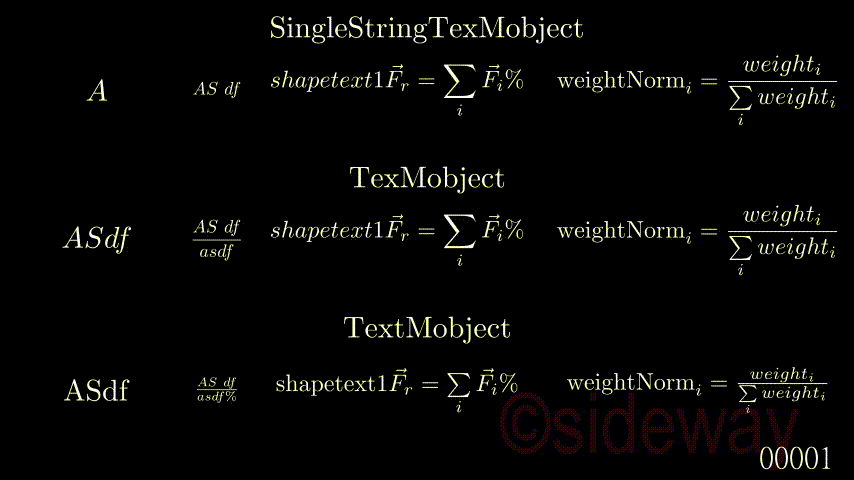output.to from Sideway

ManimKnowledge Base

tex_mobject.pyTexsymbol, SingleStringTexMobject, TexMobject, TextMobjectTexMobjectFromPresetString, BulletedList, Title

Draft for Information Only

# Content

TexMobject Class TexSymbol(VMobjectFromSVGPathstring) Class SingleStringTexMobject(SVGMobject) Class TexMobject(SVGMobject) Class TextMobject(SVGMobject)  Example   Code   Output

# TexMobject

The defined texmobject SVGMobject in tex_mobject.py
• Class TexSymbol(VMobjectFromSVGPathstring)
• Class SingleStringTexMobject(SVGMobject)
• Class TexMobject(SingleStringTexMobject)
• Class TextMobject(TexMobject)
• Class BulletedList(TextMobject)
• Class TexMobjectFromPresetString(TexMobject)
• Class Title(TextMobject)

## Class TexSymbol(VMobjectFromSVGPathstring)

is same as VMobjectFromSVGPathstring.

## Class SingleStringTexMobject(SVGMobject)

SingleStringTexMobject is used to create single string texmobject.

## Class TexMobject(SVGMobject)

TexMobject is used to create texmobject with equation is raw strings with an r preceding the quotes.

## Class TextMobject(SVGMobject)

TextMobject is used to create textmobject with equation is enclosed by a pair of dollar signs.

### Example

#### Code

# folder/file: tut/manim_mobject_singlestringtexmobjecttexmobjecttextmobject_001a.py
from manimlib.scene.scene import Scene
from manimlib.animation.creation import ShowCreation
from manimlib.mobject.svg.tex_mobject import SingleStringTexMobject, TexMobject, TextMobject
from manimlib.animation.composition import AnimationGroup

class manim_mobject_singlestringtexmobjecttexmobjecttextmobject_001a(Scene):
def construct(self):
a1=SingleStringTexMobject("A").move_to([-5.5,2.5,0])
a2=SingleStringTexMobject("AS\\ df").scale(0.6).move_to([-3.5,2.5,0])
a3=SingleStringTexMobject(r"shapetext1 \vec{F}_{r} = \sum_i \vec{F}_i \text{\%}").scale(0.8).move_to([-0.5,2.5,0])
a4=SingleStringTexMobject(r"\text{weightNorm}_i = \frac{weight_i}{\sum\limits_i weight_i}").scale(0.8).move_to([4.5,2.5,0])
b1=TexMobject("A","S","d","f").move_to([-5.5,0,0])
b2=TexMobject("AS\\ df","\\over","asdf",r"\\%").scale(0.6).move_to([-3.5,0,0])
b3=TexMobject("shapetext1",r"\vec{F}_{r} = \sum_i \vec{F}_i",r"\text{\%}").scale(0.8).move_to([-0.5,0,0])
b4=TexMobject(r"\text{weightNorm}_i = \frac{weight_i}{\sum\limits_i weight_i}").scale(0.8).move_to([4.5,0,0])
c1=TextMobject("A","S","d","f").move_to([-5.5,-2.5,0])
c2=TextMobject("$AS\\ df \\over asdf\\%$").scale(0.6).move_to([-3.5,-2.5,0])
c3=TextMobject("shapetext1","$\\vec{F}_{r} = \\sum\\limits_i \\vec{F}_i$","\\%").scale(0.8).move_to([-0.5,-2.5,0])
c4=TextMobject("$\\text{weightNorm}_i = \\frac{weight_i}{\\sum\\limits_i weight_i}$").scale(0.8).move_to([4.5,-2.5,0])
self.wait(3)
self.play(AnimationGroup(ShowCreation(a1),ShowCreation(b1),ShowCreation(c1)),run_time=5)
self.wait(3)
self.play(AnimationGroup(ShowCreation(a2),ShowCreation(b2),ShowCreation(c2)),run_time=5)
self.wait(3)
self.play(AnimationGroup(ShowCreation(a3),ShowCreation(b3),ShowCreation(c3)),run_time=5)
self.wait(3)
self.play(AnimationGroup(ShowCreation(a4),ShowCreation(b4),ShowCreation(c4)),run_time=5)
self.wait(3)

#### Outputv>

ID: 201200027 Last Updated: 27/6/2020 Revision: 0Home 5

Management

HBR 3

Information

Recreation

Culture

Chinese 1097

English 337

Computer

Hardware 149

Software

Application 198

Digitization 105

Numeric 19

Programming

Web 283

Unicode 494

HTML 65

CSS 58

ASP.NET 62

OS 389

Python 15

Knowledge

Mathematics

Algebra 25

Geometry 18

Calculus 67

Engineering

Mechanical

Rigid Bodies

Statics 92

Dynamics 37

Control

Physics

Electric 27